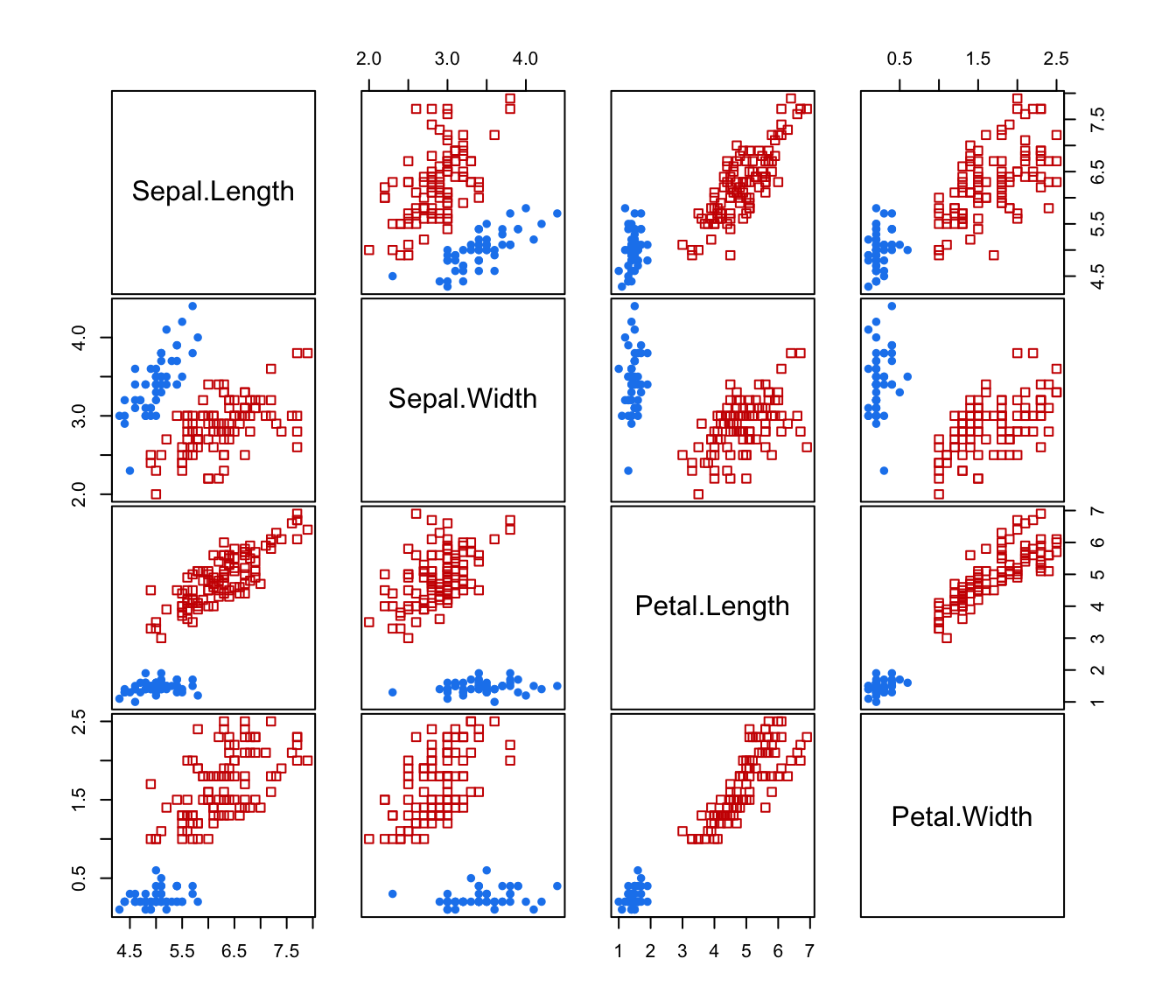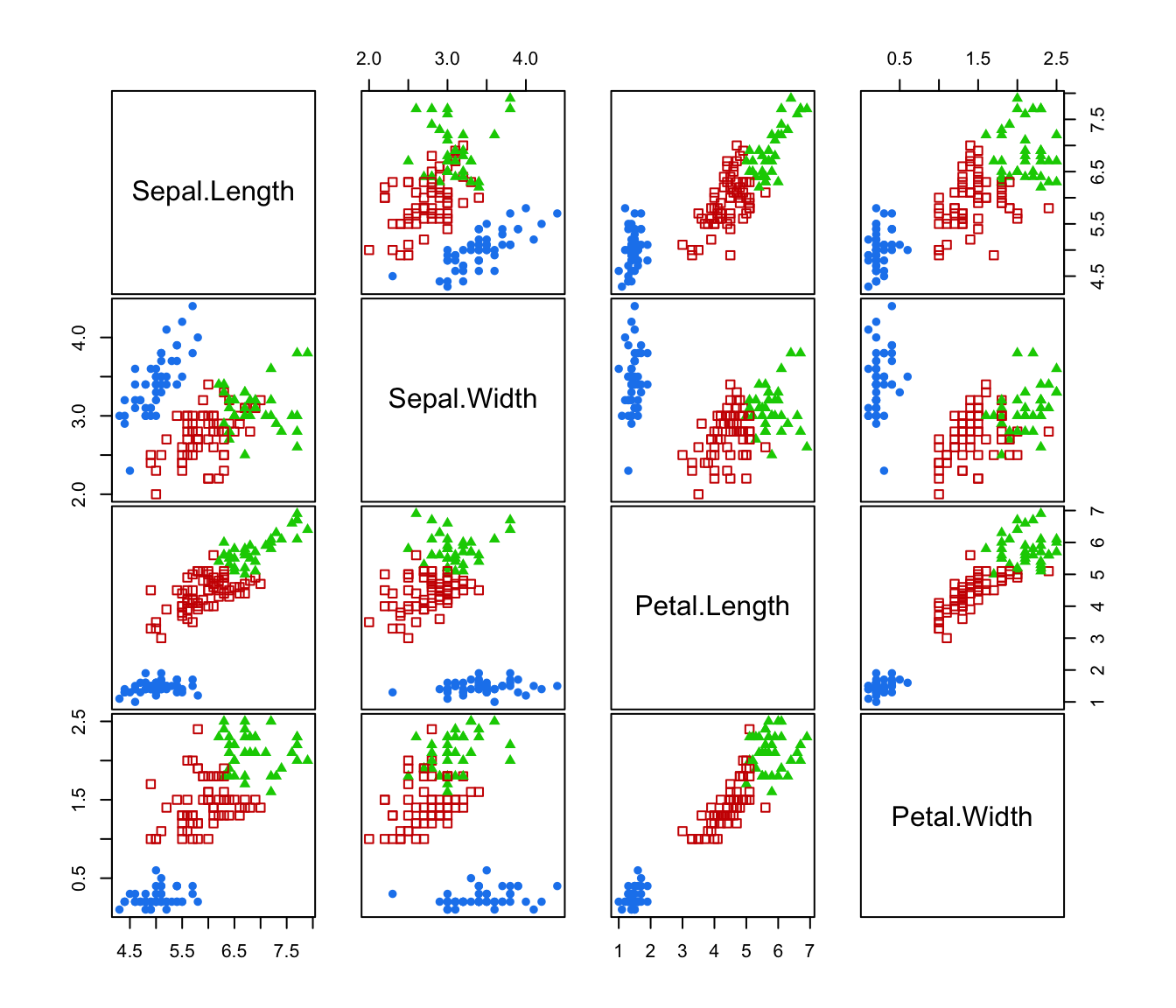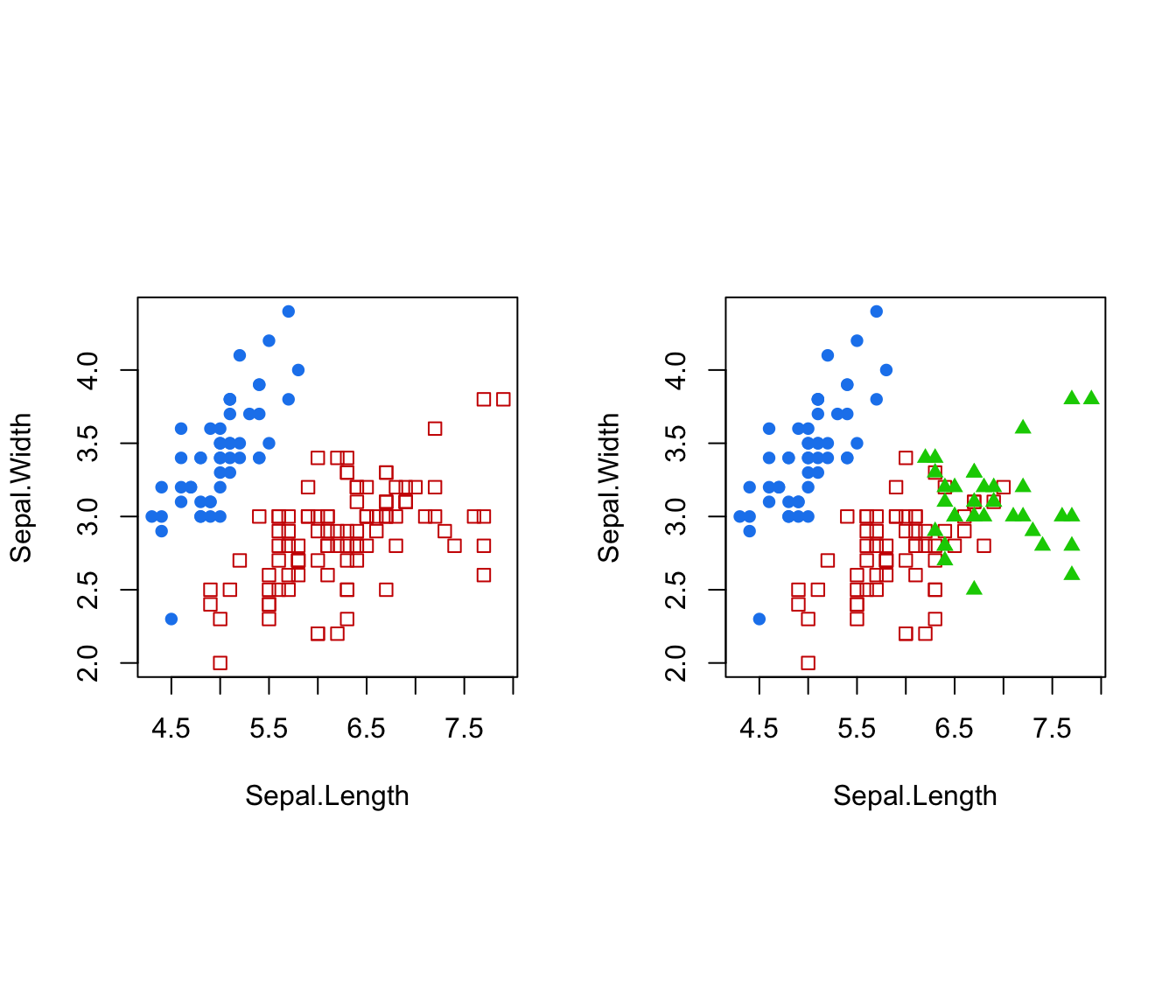Agglomerative hierarchical clustering based on maximum likelihood for a Gaussian mixture model parameterized by eigenvalue decomposition.

hcE(data, partition = NULL, minclus=1, ...)
hcV(data, partition = NULL, minclus = 1, alpha = 1, ...)
hcEII(data, partition = NULL, minclus = 1, ...)
hcVII(data, partition = NULL, minclus = 1, alpha = 1, ...)
hcEEE(data, partition = NULL, minclus = 1, ...)
hcVVV(data, partition = NULL, minclus = 1, alpha = 1, beta = 1, ...)

Arguments

data

A numeric vector, matrix, or data frame of observations. Categorical variables are not allowed. If a matrix or data frame, rows correspond to observations and columns correspond to variables.

partition

A numeric or character vector representing a partition of observations (rows) of data. If provided, group merges will start with this partition. Otherwise, each observation is assumed to be in a cluster by itself at the start of agglomeration.

minclus

A number indicating the number of clusters at which to stop the agglomeration. The default is to stop when all observations have been merged into a single cluster.

alpha, beta

Additional tuning parameters needed for initializatiion in some models. For details, see Fraley 1998. The defaults provided are usually adequate.

...

Catch unused arguments from a do.call call.

Value

A numeric two-column matrix in which the ith row gives the minimum index for observations in each of the two clusters merged at the ith stage of agglomerative hierarchical clustering.

Details

Most models have memory usage of the order of the square of the number groups in the initial partition for fast execution. Some models, such as equal variance or "EEE", do not admit a fast algorithm under the usual agglomerative hierachical clustering paradigm. These use less memory but are much slower to execute.

Examples

hcTree <- hcEII(data = iris[,-5])
cl <- hclass(hcTree,c(2,3))

# \donttest{
par(pty = "s", mfrow = c(1,1))
clPairs(iris[,-5],cl=cl[,"2"])clPairs(iris[,-5],cl=cl[,"3"])par(mfrow = c(1,2))
dimens <- c(1,2)
coordProj(iris[,-5], classification=cl[,"2"], dimens=dimens)
coordProj(iris[,-5], classification=cl[,"3"], dimens=dimens)# }